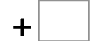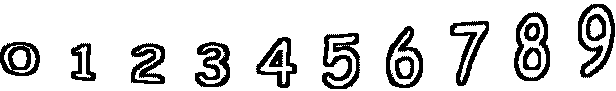0= 9   9= 95= 15   10= 19   5= 15   4= 12 3= 6   5= 11   10= 11   8= 12